# Merge Sort

Like QuickSort, Merge Sort is a Divide and Conquer algorithm. It divides input array in two halves, calls itself for the two halves and then merges the two sorted halves. The merge() function is used for merging two halves. The merge(arr, l, m, r) is key process that assumes that arr[l..m] and arr[m+1..r] are sorted and merges the two sorted sub-arrays into one. See following C implementation for details.

```MergeSort(arr[], l,  r)
If r > l
1. Find the middle point to divide the array into two halves:
middle m = (l+r)/2
2. Call mergeSort for first half:
Call mergeSort(arr, l, m)
3. Call mergeSort for second half:
Call mergeSort(arr, m+1, r)
4. Merge the two halves sorted in step 2 and 3:
Call merge(arr, l, m, r)```

The following diagram from wikipedia shows the complete merge sort process for an example array {38, 27, 43, 3, 9, 82, 10}. If we take a closer look at the diagram, we can see that the array is recursively divided in two halves till the size becomes 1. Once the size becomes 1, the merge processes comes into action and starts merging arrays back till the complete array is merged.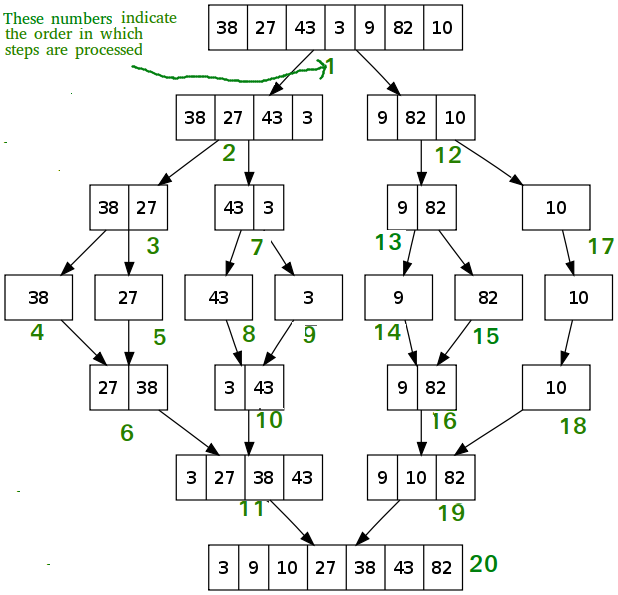## C/C++

 `/* C program for Merge Sort */` `#include ` `#include ` ` `  `// Merges two subarrays of arr[]. ` `// First subarray is arr[l..m] ` `// Second subarray is arr[m+1..r] ` `void` `merge(``int` `arr[], ``int` `l, ``int` `m, ``int` `r) ` `{ ` `    ``int` `i, j, k; ` `    ``int` `n1 = m - l + 1; ` `    ``int` `n2 = r - m; ` ` `  `    ``/* create temp arrays */` `    ``int` `L[n1], R[n2]; ` ` `  `    ``/* Copy data to temp arrays L[] and R[] */` `    ``for` `(i = 0; i < n1; i++) ` `        ``L[i] = arr[l + i]; ` `    ``for` `(j = 0; j < n2; j++) ` `        ``R[j] = arr[m + 1 + j]; ` ` `  `    ``/* Merge the temp arrays back into arr[l..r]*/` `    ``i = 0; ``// Initial index of first subarray ` `    ``j = 0; ``// Initial index of second subarray ` `    ``k = l; ``// Initial index of merged subarray ` `    ``while` `(i < n1 && j < n2) { ` `        ``if` `(L[i] <= R[j]) { ` `            ``arr[k] = L[i]; ` `            ``i++; ` `        ``} ` `        ``else` `{ ` `            ``arr[k] = R[j]; ` `            ``j++; ` `        ``} ` `        ``k++; ` `    ``} ` ` `  `    ``/* Copy the remaining elements of L[], if there ` `       ``are any */` `    ``while` `(i < n1) { ` `        ``arr[k] = L[i]; ` `        ``i++; ` `        ``k++; ` `    ``} ` ` `  `    ``/* Copy the remaining elements of R[], if there ` `       ``are any */` `    ``while` `(j < n2) { ` `        ``arr[k] = R[j]; ` `        ``j++; ` `        ``k++; ` `    ``} ` `} ` ` `  `/* l is for left index and r is right index of the ` `   ``sub-array of arr to be sorted */` `void` `mergeSort(``int` `arr[], ``int` `l, ``int` `r) ` `{ ` `    ``if` `(l < r) { ` `        ``// Same as (l+r)/2, but avoids overflow for ` `        ``// large l and h ` `        ``int` `m = l + (r - l) / 2; ` ` `  `        ``// Sort first and second halves ` `        ``mergeSort(arr, l, m); ` `        ``mergeSort(arr, m + 1, r); ` ` `  `        ``merge(arr, l, m, r); ` `    ``} ` `} ` ` `  `/* UTILITY FUNCTIONS */` `/* Function to print an array */` `void` `printArray(``int` `A[], ``int` `size) ` `{ ` `    ``int` `i; ` `    ``for` `(i = 0; i < size; i++) ` `        ``printf``(``"%d "``, A[i]); ` `    ``printf``(``"\n"``); ` `} ` ` `  `/* Driver program to test above functions */` `int` `main() ` `{ ` `    ``int` `arr[] = { 12, 11, 13, 5, 6, 7 }; ` `    ``int` `arr_size = ``sizeof``(arr) / ``sizeof``(arr); ` ` `  `    ``printf``(``"Given array is \n"``); ` `    ``printArray(arr, arr_size); ` ` `  `    ``mergeSort(arr, 0, arr_size - 1); ` ` `  `    ``printf``(``"\nSorted array is \n"``); ` `    ``printArray(arr, arr_size); ` `    ``return` `0; ` `} `

## Java

 `/* Java program for Merge Sort */` `class` `MergeSort { ` `    ``// Merges two subarrays of arr[]. ` `    ``// First subarray is arr[l..m] ` `    ``// Second subarray is arr[m+1..r] ` `    ``void` `merge(``int` `arr[], ``int` `l, ``int` `m, ``int` `r) ` `    ``{ ` `        ``// Find sizes of two subarrays to be merged ` `        ``int` `n1 = m - l + ``1``; ` `        ``int` `n2 = r - m; ` ` `  `        ``/* Create temp arrays */` `        ``int` `L[] = ``new` `int``[n1]; ` `        ``int` `R[] = ``new` `int``[n2]; ` ` `  `        ``/*Copy data to temp arrays*/` `        ``for` `(``int` `i = ``0``; i < n1; ++i) ` `            ``L[i] = arr[l + i]; ` `        ``for` `(``int` `j = ``0``; j < n2; ++j) ` `            ``R[j] = arr[m + ``1` `+ j]; ` ` `  `        ``/* Merge the temp arrays */` ` `  `        ``// Initial indexes of first and second subarrays ` `        ``int` `i = ``0``, j = ``0``; ` ` `  `        ``// Initial index of merged subarry array ` `        ``int` `k = l; ` `        ``while` `(i < n1 && j < n2) { ` `            ``if` `(L[i] <= R[j]) { ` `                ``arr[k] = L[i]; ` `                ``i++; ` `            ``} ` `            ``else` `{ ` `                ``arr[k] = R[j]; ` `                ``j++; ` `            ``} ` `            ``k++; ` `        ``} ` ` `  `        ``/* Copy remaining elements of L[] if any */` `        ``while` `(i < n1) { ` `            ``arr[k] = L[i]; ` `            ``i++; ` `            ``k++; ` `        ``} ` ` `  `        ``/* Copy remaining elements of R[] if any */` `        ``while` `(j < n2) { ` `            ``arr[k] = R[j]; ` `            ``j++; ` `            ``k++; ` `        ``} ` `    ``} ` ` `  `    ``// Main function that sorts arr[l..r] using ` `    ``// merge() ` `    ``void` `sort(``int` `arr[], ``int` `l, ``int` `r) ` `    ``{ ` `        ``if` `(l < r) { ` `            ``// Find the middle point ` `            ``int` `m = (l + r) / ``2``; ` ` `  `            ``// Sort first and second halves ` `            ``sort(arr, l, m); ` `            ``sort(arr, m + ``1``, r); ` ` `  `            ``// Merge the sorted halves ` `            ``merge(arr, l, m, r); ` `        ``} ` `    ``} ` ` `  `    ``/* A utility function to print array of size n */` `    ``static` `void` `printArray(``int` `arr[]) ` `    ``{ ` `        ``int` `n = arr.length; ` `        ``for` `(``int` `i = ``0``; i < n; ++i) ` `            ``System.out.print(arr[i] + ``" "``); ` `        ``System.out.println(); ` `    ``} ` ` `  `    ``// Driver method ` `    ``public` `static` `void` `main(String args[]) ` `    ``{ ` `        ``int` `arr[] = { ``12``, ``11``, ``13``, ``5``, ``6``, ``7` `}; ` ` `  `        ``System.out.println(``"Given Array"``); ` `        ``printArray(arr); ` ` `  `        ``MergeSort ob = ``new` `MergeSort(); ` `        ``ob.sort(arr, ``0``, arr.length - ``1``); ` ` `  `        ``System.out.println(``"\nSorted array"``); ` `        ``printArray(arr); ` `    ``} ` `} ` `/* This code is contributed by Rajat Mishra */`

## Python3

 `# Python program for implementation of MergeSort ` `def` `mergeSort(arr): ` `    ``if` `len``(arr) >``1``: ` `        ``mid ``=` `len``(arr)``/``/``2` `# Finding the mid of the array ` `        ``L ``=` `arr[:mid] ``# Dividing the array elements  ` `        ``R ``=` `arr[mid:] ``# into 2 halves ` ` `  `        ``mergeSort(L) ``# Sorting the first half ` `        ``mergeSort(R) ``# Sorting the second half ` ` `  `        ``i ``=` `j ``=` `k ``=` `0` `         `  `        ``# Copy data to temp arrays L[] and R[] ` `        ``while` `i < ``len``(L) ``and` `j < ``len``(R): ` `            ``if` `L[i] < R[j]: ` `                ``arr[k] ``=` `L[i] ` `                ``i``+``=` `1` `            ``else``: ` `                ``arr[k] ``=` `R[j] ` `                ``j``+``=` `1` `            ``k``+``=` `1` `         `  `        ``# Checking if any element was left ` `        ``while` `i < ``len``(L): ` `            ``arr[k] ``=` `L[i] ` `            ``i``+``=` `1` `            ``k``+``=` `1` `         `  `        ``while` `j < ``len``(R): ` `            ``arr[k] ``=` `R[j] ` `            ``j``+``=` `1` `            ``k``+``=` `1` ` `  `# Code to print the list ` `def` `printList(arr): ` `    ``for` `i ``in` `range``(``len``(arr)):         ` `        ``print``(arr[i], end ``=``" "``) ` `    ``print``() ` ` `  `# driver code to test the above code ` `if` `__name__ ``=``=` `'__main__'``: ` `    ``arr ``=` `[``12``, ``11``, ``13``, ``5``, ``6``, ``7``]  ` `    ``print` `(``"Given array is"``, end ``=``"\n"``)  ` `    ``printList(arr) ` `    ``mergeSort(arr) ` `    ``print``(``"Sorted array is: "``, end ``=``"\n"``) ` `    ``printList(arr) ` ` `  `# This code is contributed by Mayank Khanna `

## Python (Alternative)

 `# Python program for implementation of  ` `# MergeSort (Alternative) ` ` `  `def` `merge_sort(values): ` ` `  `    ``if` `len``(values)>``1``: ` `        ``m ``=` `len``(values)``/``/``2` `        ``left ``=` `values[:m] ` `        ``right ``=` `values[m:] ` `        ``left ``=` `merge_sort(left) ` `        ``right ``=` `merge_sort(right) ` ` `  `        ``values ``=``[] ` ` `  `        ``while` `len``(left)>``0` `and` `len``(right)>``0``: ` `            ``if` `left[``0``]

Output:

```Given array is
12 11 13 5 6 7

Sorted array is
5 6 7 11 12 13
```

Time Complexity: Sorting arrays on different machines. Merge Sort is a recursive algorithm and time complexity can be expressed as following recurrence relation.
T(n) = 2T(n/2) +The above recurrence can be solved either using Recurrence Tree method or Master method. It falls in case II of Master Method and solution of the recurrence is.
Time complexity of Merge Sort isin all 3 cases (worst, average and best) as merge sort always divides the array into two halves and take linear time to merge two halves.

Auxiliary Space: O(n)

Sorting In Place: No in a typical implementation

Stable: Yes

Applications of Merge Sort

1. Merge Sort is useful for sorting linked lists in O(nLogn) time.In the case of linked lists, the case is different mainly due to the difference in memory allocation of arrays and linked lists. Unlike arrays, linked list nodes may not be adjacent in memory. Unlike an array, in the linked list, we can insert items in the middle in O(1) extra space and O(1) time. Therefore merge operation of merge sort can be implemented without extra space for linked lists.

In arrays, we can do random access as elements are contiguous in memory. Let us say we have an integer (4-byte) array A and let the address of A be x then to access A[i], we can directly access the memory at (x + i*4). Unlike arrays, we can not do random access in the linked list. Quick Sort requires a lot of this kind of access. In linked list to access i’th index, we have to travel each and every node from the head to i’th node as we don’t have a continuous block of memory. Therefore, the overhead increases for quicksort. Merge sort accesses data sequentially and the need of random access is low.

2. Inversion Count Problem
3. Used in External Sorting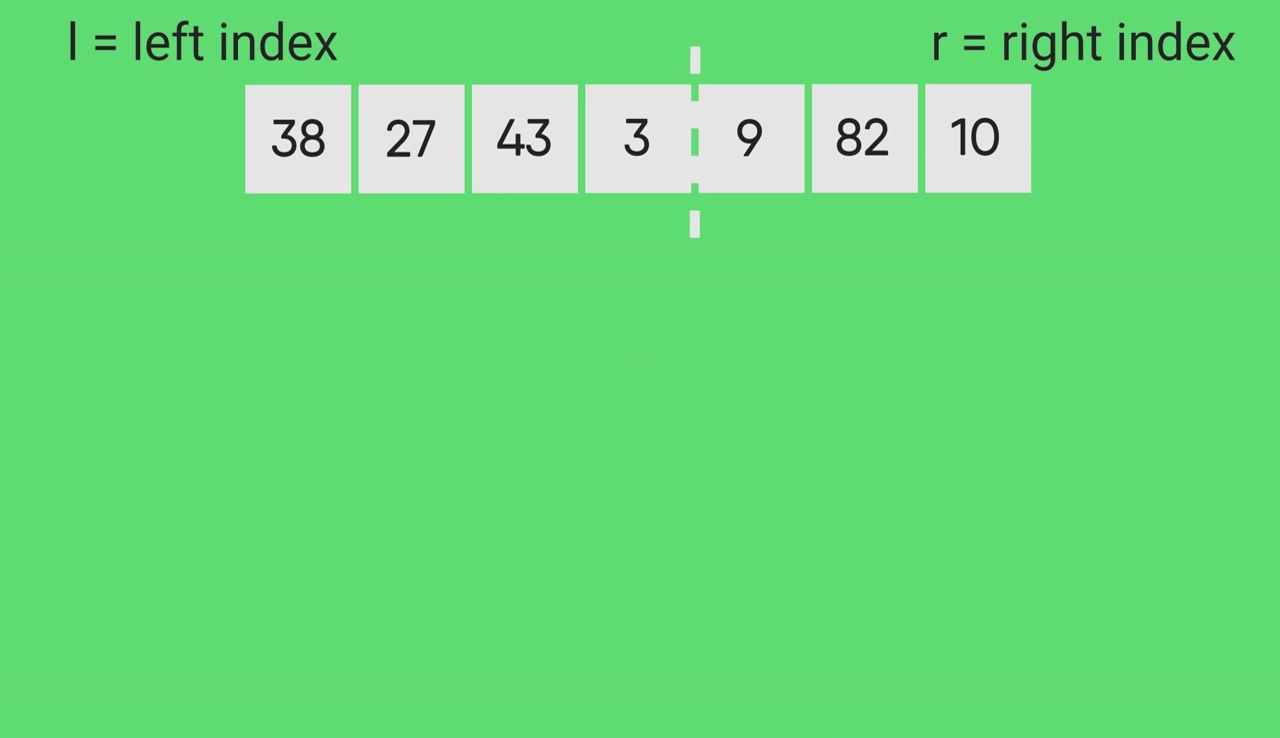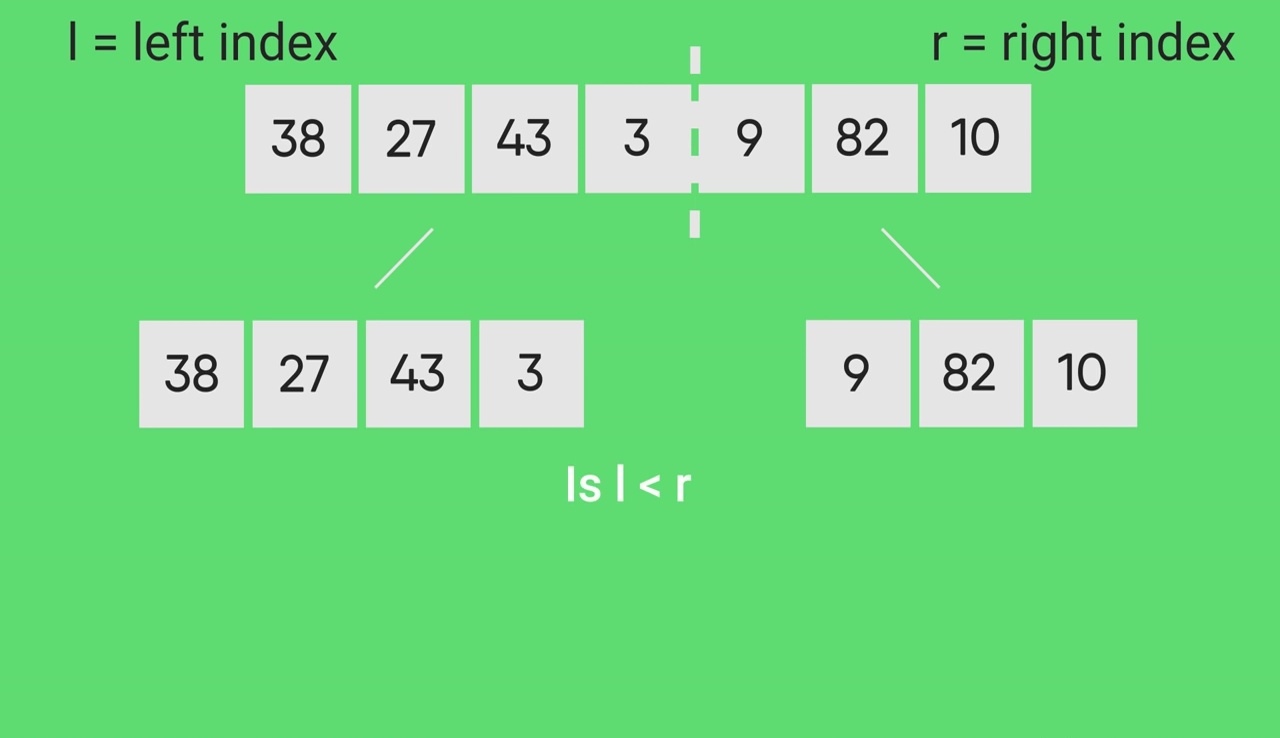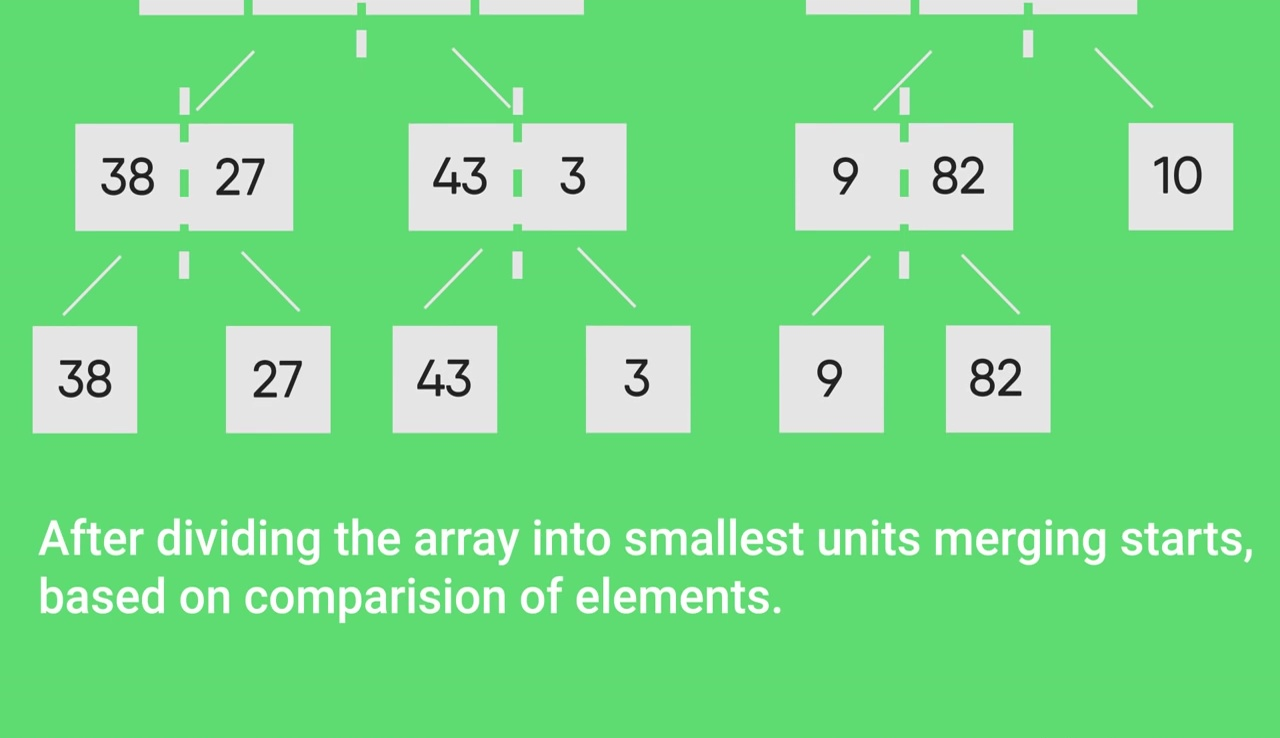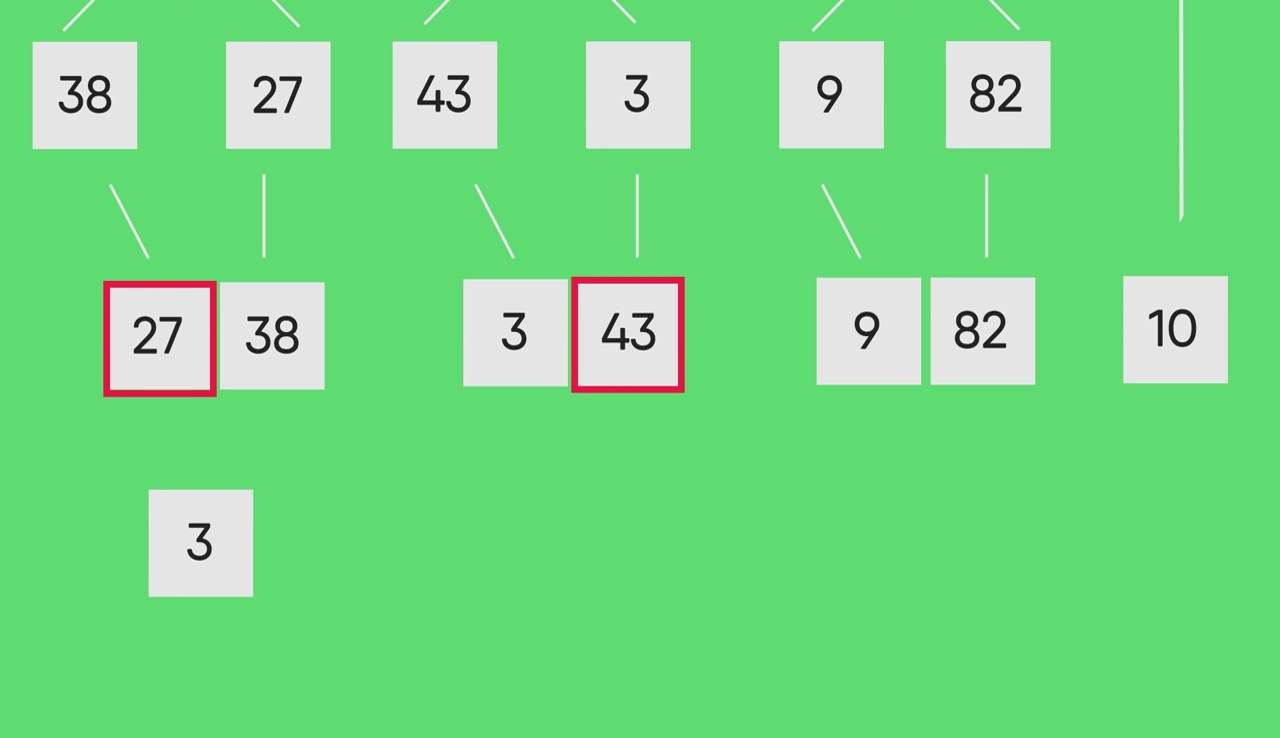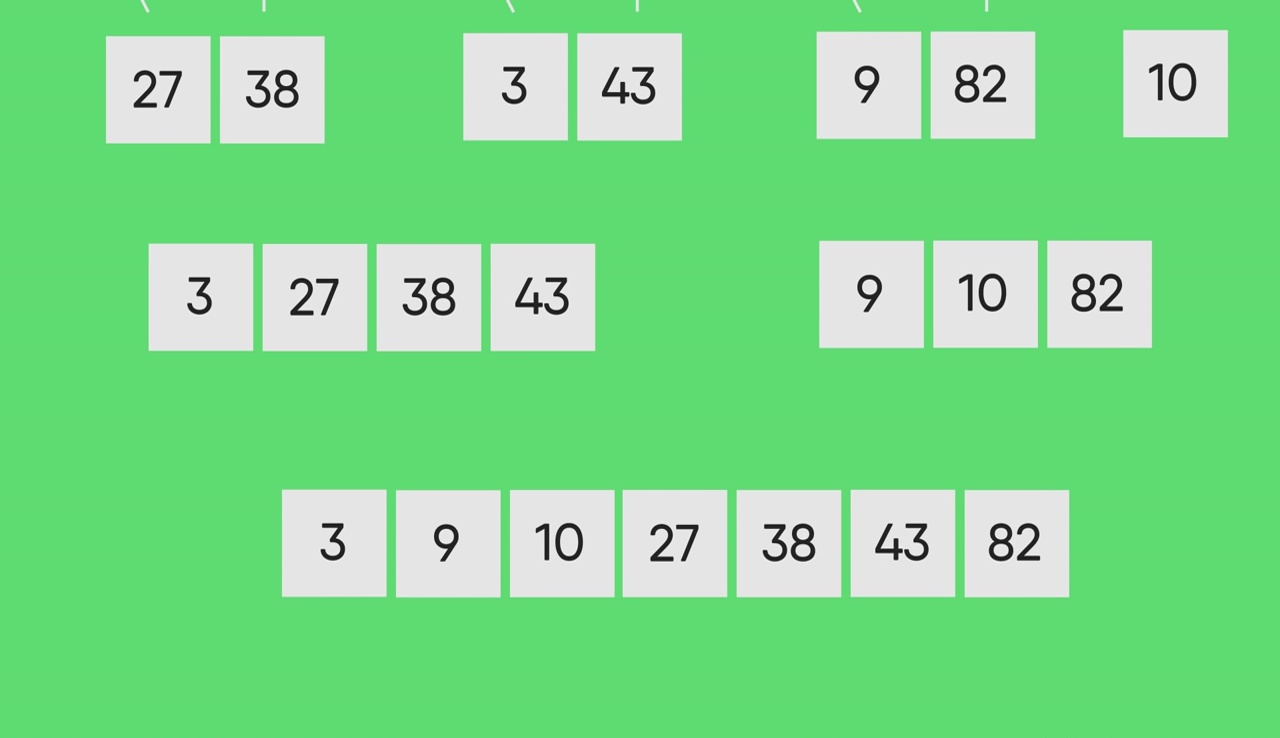Other Sorting Algorithms on GeeksforGeeks:
3-way Merge Sort, Selection Sort, Bubble Sort, Insertion Sort, Merge Sort, Heap Sort, QuickSort, Radix Sort, Counting Sort, Bucket Sort, ShellSort, Comb Sort

Don’t stop now and take your learning to the next level. Learn all the important concepts of Data Structures and Algorithms with the help of the most trusted course: DSA Self Paced. Become industry ready at a student-friendly price.

My Personal Notes arrow_drop_up

Article Tags :

188

Please write to us at contribute@geeksforgeeks.org to report any issue with the above content.